# Kernel Density Estimation (KDE) Plot

Previously, we saw how to use the histogram method to infer the probability density function (PDF) of a random variable (for example, population) using a finite data sample. In this tutorial, we’ll carry on the problem of probability density function inference but using another method: Kernel density estimation (KDE).

Kernel density estimates are closely related to histograms but can be endowed with properties such as smoothness or continuity by using a suitable kernel.

## Process

Using NumXL, you can compute the KDE values for different kernel functions (e.g., Gaussian, uniform, triangular, quartic, triweight, cosine, etc.) and (optionally) with a bandwidth value.

For our sample data, we are using 100 randomly generated values of the normal distribution (using the random generator in the Excel Analysis Pack). We plotted the histogram for our reference: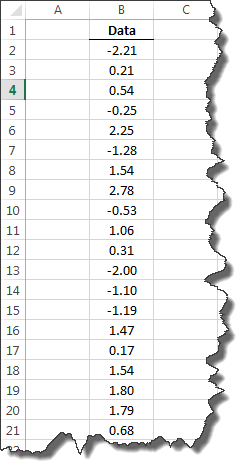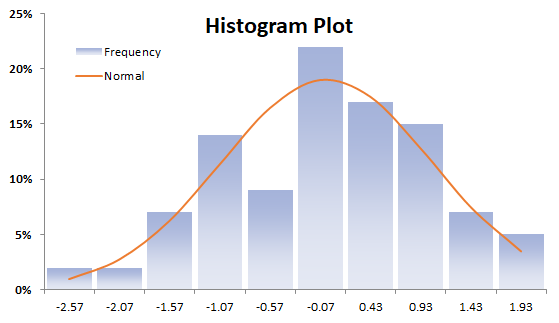Now we are ready to construct our KDE plot. First, select the empty cell in your worksheet where you wish to generate the output, then locate and click on the Descriptive Statistics icon in the NumXL tab (or toolbar). Then, select the Kernel Density Estimation (KDE) item from the drop-down menu.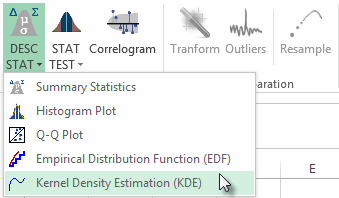The KDE wizard appears.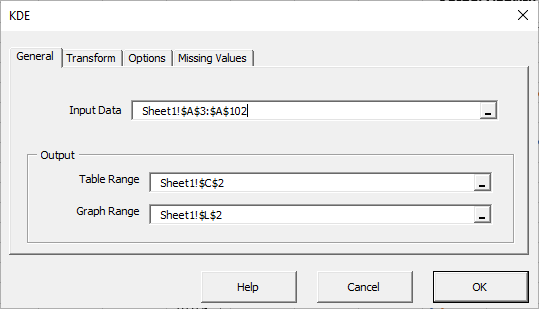In the Input Data field, select the cells range for the values of the input variable.

Notes:

1. The cell range includes (optional) the heading (Label) cell, which would be used in the output tables where it references those variables.
2. By default, the output table cells range is set to the currently selected cell in your worksheet.
3. By default, the output graph cells range is set to the 7 cells to the right of the currently selected cell in your worksheet.

Once we select the input data (X) cells range, the Transform, Options, and Missing Values tabs become available (enabled).

Are the input data values bound by an upper or lower limit? If so, enter those limits and, optionally, a transform function to enforce them.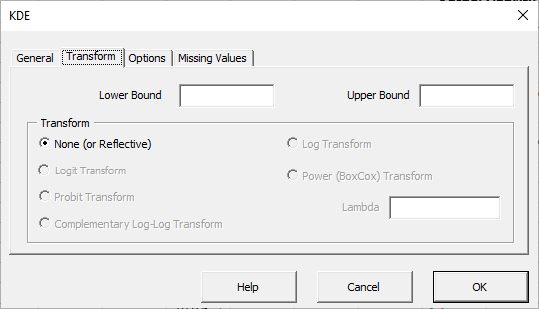For our data set, there is no lower or upper bound, so we’ll leave the fields in the Transform tab empty or blank.

Next, select the Options tab: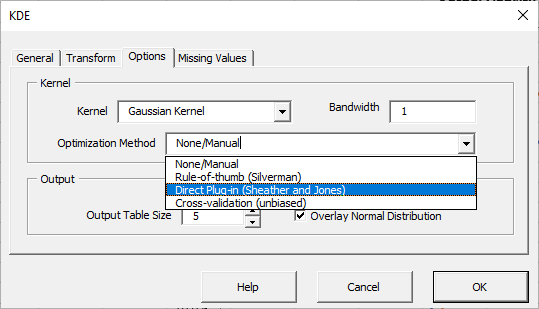Notes:

1. By default, the Gaussian kernel function is selected. Let’s leave this option unchanged.
2. By default, the Optimization Method dropdown is set to None/Manual. Change it to Direct Plug-in (Sheather & Jones).
3. By default, the output table size is set to 5. Change it to 15.
4. “Overlay Normal distribution” is checked. This option in effect instructs the wizard to generate a second curve for the Gaussian distribution for comparison purposes. Leave this option checked.

Next, click the “Missing Values” tab.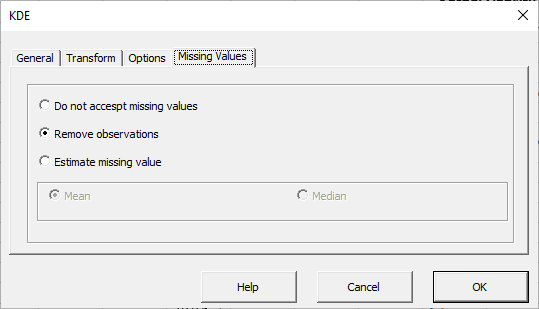In this tab, you can select an approach to handle missing values in the data set (X’s). By default, any observation with missing values would be excluded from the analysis.

This treatment is a good approach for our analysis, so let’s leave it unchanged.

Now, click OK to generate the output tables.

## Output

Case 1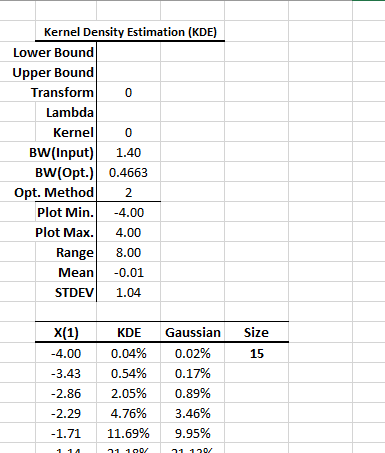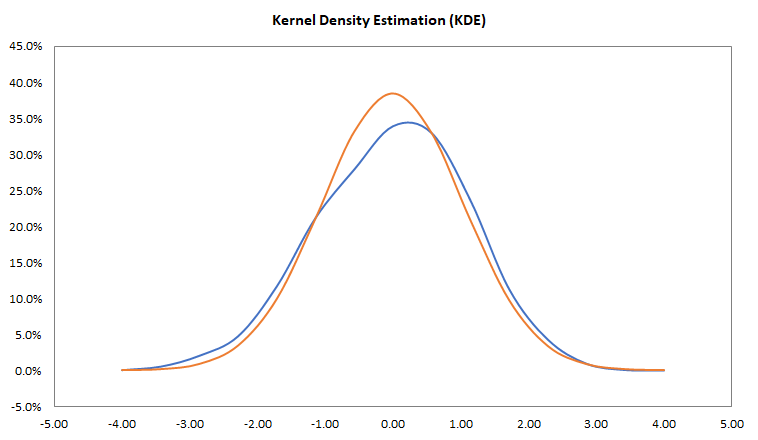Notes:

1. The upper part of the output lists the parameters’ values of the NxKDE(.) function. You may change those settings.
2. The Plot Min. and Plot Max. cells define the x-axis limits in the plot, so you can change their values to examine different parts of the KDE. In this tutorial, we’ll view the KDE in a (-4.0, 4.0) interval.
3. There are two entries for bandwidth: BW (input) and BW (Opt.). You may enter any value in the BW (input), but the BW (Opt.) is calculated using the NxKDE(.) Function. For the Manual Bandwith method, the BW (Opt.) is equal to BW(Input), and for all others, the BW(Input) serves as an initial value.
4. The values of all X are sorted in ascending order.
5. The NxKDE(.) supports 7 different kernel functions: Gaussian, uniform, triangular, quartic, tri-weight, Epanechnikov, and cosine functions.
6. The Direct Plug-in (DPI) optimization method requires a differentiable kernel function up to the 6th order, so only Gaussian and cosine kernels can be used with DPI.

Note that the KDE curve (blue) tracks very closely with the Gaussian density (orange) curve.

From the output table, let’s change the optimization method to 3, which corresponds to the unbiased cross-validation method, and observe the recalculation of the optimal bandwidth and KDE values.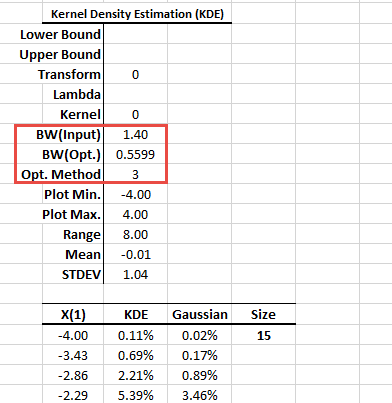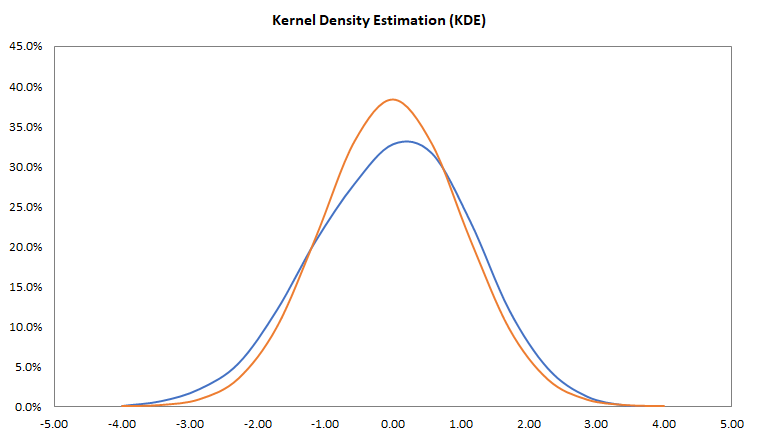Case 2

Now let’s try a non-normal sample data set. We generated 100 random values of a uniform distribution between -3 and 3. Following similar steps, we plotted the histogram and the KDE: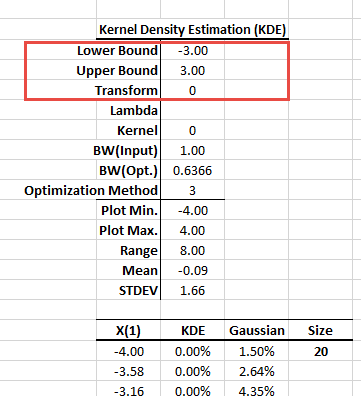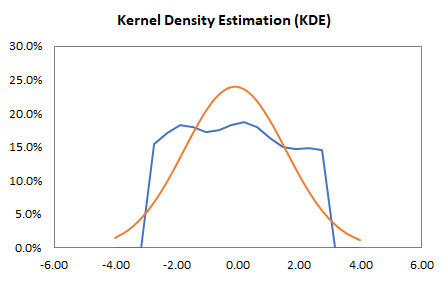Note that we entered lower and upper bounds to the KDE, but did not specify a transform function. In this case, the NxKDE(.) reverts to Silverman’s reflection method for those values near the domain bounds.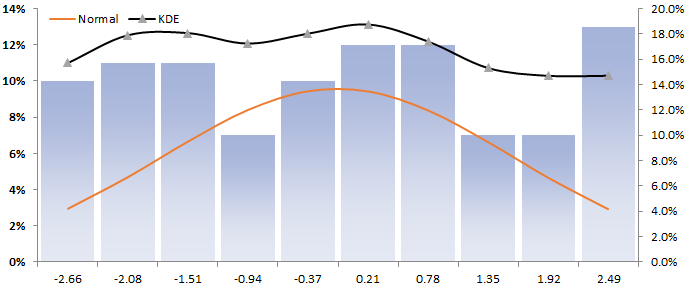Note that the KDE curve (black) tracks more closely with the underlying distribution (i.e., uniform) than the histogram or the normal curve.

## Conclusion

In this tutorial, we demonstrated the process to generate a KDE in Excel using NumXL’s add-in functions.

The KDE method is a major improvement for inferring the probability density function of the population, in terms of accuracy and continuity of the function.

We have used the NxKDE function to calculate the optimal bandwidth and the probability density values. The NxKDE(.) function supports additional output values: cumulative density function (CDF) and its inverse (inverse CDF).